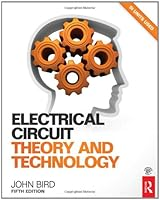# Electrical Circuit Theory and Technology, 5th Edition1 Reviews
2013-12-14
784 pages

## Book Description

This much-loved textbook explains the of electrical circuit theory and technology so that students of electrical and can master the subject.

Real-world situations and examples put the theory into context. The inclusion of worked problems with solutions help you to learn and further problems then allow you to test and confirm you have fully understood each subject. In total the book contains 800 worked problems, 1000 further problems and 14 revision tests with answers online.

This an ideal text for foundation and undergraduate degree students and those on upper level vocational engineering courses, in particular electrical and mechanical. It provides a sound understanding of the knowledge required by technicians in fields such as electrical engineering, electronics and telecommunications.

This edition has been updated with developments in key areas such as semiconductors, transistors, and fuel cells, along with brand new material on ABCD parameters and Fourier’s . It is supported by a companion website that contains solutions to the 1000 questions in the practice exercises, formulae to help students answer the questions and information about the famous mathematicians and scientists mentioned in the book. Lecturers also have to full solutions and the marking scheme for the 14 revision tests, lesson plans and illustrations from the book.

Part 1 Basic electrical engineering principles
Chapter 1 Units associated with basic electrical quantities
Chapter 2 An introduction to electric circuits
Chapter 3 Resistance variation
Chapter 4 Batteries and alternative sources of energy
Chapter 5 Series and parallel networks
Chapter 6 Capacitors and capacitance
Chapter 7 Magnetic circuits
Chapter 8 Electromagnetism
Chapter 9 Electromagnetic induction
Chapter 10 Electrical measuring instruments and measurements
Chapter 11 Semiconductor diodes
Chapter 12 Transistors

Part 2 Electrical principles and technology
Chapter 13 D.c. circuit theory
Chapter 14 Alternating voltages and currents
Chapter 15 Single-phase series a.c. circuits
Chapter 16 Single-phase parallel a.c. circuits
Chapter 17 D.c. transients
Chapter 18 Operational amplifiers
Chapter 19 Three-phase
Chapter 20 Transformers
Chapter 21 D.c. machines
Chapter 22 Three-phase induction motors

Part 3 Advanced circuit theory and technology
Chapter 23 Revision of complex numbers
Chapter 24 Application of complex numbers to series a.c. circuits
Chapter 25 Application of complex numbers to parallel a.c. networks
Chapter 26 Power in a.c. circuits
Chapter 27 A.c. bridges
Chapter 28 Series resonance and Q-factor
Chapter 29 Parallel resonance and Q-factor
Chapter 30 Introduction to network analysis
Chapter 31 Mesh-current and nodal analysis
Chapter 32 The superposition theorem
Chapter 33 Thévenin’s and Norton’s theorems
Chapter 34 Delta–star and star–delta transformations
Chapter 35 Maximum power transfer theorems and impedance matching
Chapter 36 Complex waveforms
Chapter 37 A numerical method of harmonic analysis
Chapter 38 Magnetic materials
Chapter 39 Dielectrics and dielectric loss
Chapter 40 Field theory
Chapter 41 Attenuators
Chapter 42 Filter networks
Chapter 43 Magnetically coupled circuits
Chapter 44 Transmission lines
Chapter 45 Transients and Laplace transforms

Part 4 General reference

## Book Details

• Title: Electrical Circuit Theory and Technology, 5th Edition
• Author:
• Length: 784 pages
• Edition: 5
• Language: English
• Publisher:
• Publication Date: 2013-12-14
• ISBN-10: 0415662869
• ISBN-13: 9781466501096